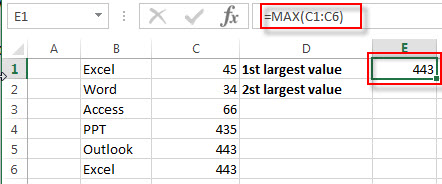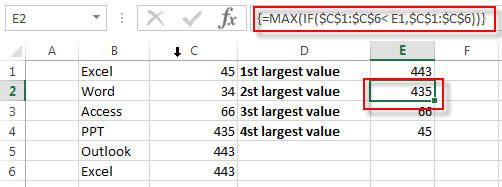# Get nth Largest Value with Duplicates

This post will discuss that how to find and get the Nth largest unique value in a range of cells in Excel. Normally, you can use the LARGE function to get the first or second or nth largest value, but it cannot be filtered the duplicated values.

## Get nth Largest Value with Duplicates

If you want to get the largest unique value in a data set with duplicates, you can create a new excel array formula based on the MAX function and the IF function.

Assuming you have a data set with duplicates in a range of cells C1:C6, and you want to extract the First and second largest unique values.

 Excel 45 Word 34 Access 66 PPT 435 Outlook 443 Excel 443

To get the Nth largest values with duplicates, just do the following steps:

#1 you need to get the first largest value using MAX function. Enter the following formula into the formula box in cell E1:

`=MAX(C1:C6)`#2 you need to write down the below array formula to get the second or nth (n>1) largest unique value:

`{=MAX(IF(\$C\$1:\$C\$6< E1,\$C\$1:\$C\$6))}`

Enter this formula into the Cell E2, then press Ctrl + Shift + Enter shortcuts to get the second largest value.### Related Formulas

• Get nth Match with One Criteria using INDEX/MATCH
if you want to find the 2th occurrence of the member “jenny” in the range B2:B10 and extracts its relative bonus value in the range D2:D10, you can used the following array formula:=INDEX(D2:D10, SMALL(IF(B2:B10=”jenny”, ROW(B2:B10)-ROW(INDEX(B2:B10,1,1))+1),2))…
• Lookup the Value with Multiple Criteria
If you want to lookup the value with multiple criteria in a range, you can use a combination with the INDEX function and MATCH function to create an array formula.…
• Lookup the Next Largest Value
If you want to get the next largest value in another column, you can use a combination of the INDEX function and the MATCH function to create an excel formula..
• Find the nth Smallest Value
You can use the SMALL function to get the 1st, 2nd, 3rd, or nth smallest value in an array or range. Also you can use the SMALL function within the INDEX function to extract the relative value of the same row…

### Related Functions

• Excel LARGE function
The Excel LARGE function returns the largest numeric value from the numbers that you provided. Or returns the largest value in the array. The syntax of the LARGE function is as below:= LARGE (array,nth)…
• Excel MAX function
The Excel MAX function returns the largest numeric value from the numbers that you provided. Or returns the largest value in the array.= MAX(num1,[num2,…numn])…
• Excel IF function
The Excel IF function perform a logical test to return one value if the condition is TRUE and return another value if the condition is FALSE. The IF function is a build-in function in Microsoft Excel and it is categorized as a Logical Function.The syntax of the IF function is as below:= IF (condition, [true_value], [false_value])….